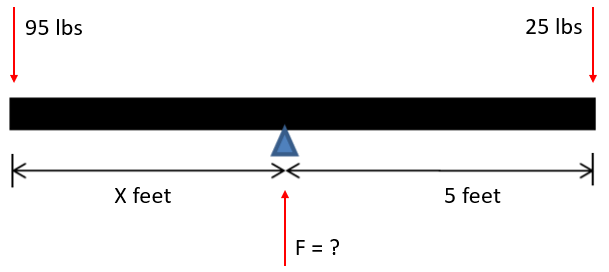## Fulcrum Force

Neglecting the beam's mass, calculate the fulcrum force applied to the beam.Hint
Since the beam is balanced, we can sum the forces in the y-direction to determine the fulcrum force.
Since the beam is balanced, we can sum the forces in the y-direction to determine the fulcrum force.
$$+ \uparrow \sum F_y=0: -95\:lbs+-25\:lbs+F=0$$$$$+ \uparrow \sum F_y=0: -120\:lbs+F=0$$$
$$+ \uparrow \sum F_y=0: F= 120\:lbs$$\$
120 lbs